Click to Chat

1800-1023-196

+91-120-4616500

CART 0

• 0

MY CART (5)

Use Coupon: CART20 and get 20% off on all online Study Material

ITEM
DETAILS
MRP
DISCOUNT
FINAL PRICE
Total Price: Rs.

There are no items in this cart.
Continue Shopping• Complete Physics Course - Class 11
• OFFERED PRICE: Rs. 2,968
• View Details

```Solved Examples on Fluid Mechanics

Problem 1:-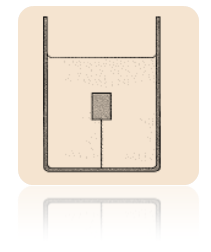The tension in a string holding a solid block below the surface of a liquid (of density greater than the solid) is T0 when the containing vessel (see below figure) is at rest. Show that the tension T, when the vessel has an upward vertical acceleration a, is given by T0 (1+a/g).

Concept:-

The various forces acting on the block when it is inside the water are the weight, the buoyant force and the tension in the string. When the vessel is at rest, there is no net force acting on the block. The sum of the three forces is equal to zero when the vessel is at rest.

When the vessel moves upward with an acceleration, the buoyant force and the tension in the string will be differ. There is a net force acting vessel. Thus, the sum of the three forces is equal to the net force acting on the block.

Solution:-

The weight of the block having mass m which is acting downward is

W = mg

Here,

Acceleration due to gravity at the point of observation is g.

The magnitude of the buoyant which acts upwards is

Fb = Vρg

Here,

Volume of the water displaced by the block is V

Density of the water is ρ.

The various forces acting on the block which is placed inside the vessel is shown below:As the vessel is rest, the three forces are in equilibrium. Thus, the sum of the three forces is equal to zero.

Thus,

ΣF = 0

This makes

W – Fb – T0 = 0

Here,

Tension in the string when the vessel is at rest is T0.

Insert the values of the various terms involved in the above equation gives

Fb – W – T0 = 0

Vρg – mg – T0 = 0

So, T0 = Vρg – mg

This represents the tension in the string when the vessel is at rest.

When the vessel is moving with an upward vertical acceleration a, there is net forces acting on the block. So, there is change in the buoyant force and the tension in the string.

The figure which shows the forces acting on the block when the vessel is accelerating upward is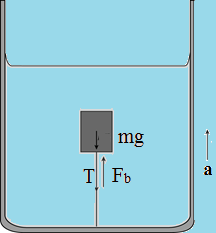The buoyant forces acting on the block is

Fb,a = Vρ (g+a)

Thus, the net forces is given as

ΣF = ma

It is equal to the sum of the buoyant force, the weight of the block and tension (T).

Thus,

Fb,a – W -T = Ma

Substitute the values of Fb,a and W  in the above equation gives

Fb,a – W – T = ma

Vρ (g+a) – mg – T = ma

So, T = Vρa +Vρg – mg – ma

To obtain the tension in the string, substitute T0 = Vρg – mg in the above equation

T = Vρg – mg + Vρa – ma = T0 + a (Vρ – m)

Dividing both numerator and denominator in the second part in the right hand side of the equation by g gives

T = T0 + [a/g (Vρg – mg)]

= T0 +[(a/g) T0] = T0 (1+a/g)

This represents the tension in the string when the vessel is accelerating upward.

_______________________________________________________________________________________________________

Problem 2:-A cubic object of dimensions L = 0.608 m on a side and weight W = 4450 N in a vacuum is suspended by a wire in an open tank of liquid of density ρ = 944 kg/m3, as shown in below figure.

(a) Find the total downward force exerted by the liquid and the atmosphere on the top of the object. (b) Find the total upward force on the bottom of the object. (c)  Find the tension in the wire. (d) Calculate the buoyant force on the object using Archimedes principle. What relation exists among all these quantities?

Concept:-

The pressure acting on the top of the object is

pT = p0 +ρg (L/2)

Here, atmospheric pressure is p0, density of the liquid is ρ, acceleration due to gravity is g and length of the side of the object is L.

The total downward force exerted by the liquid and the atmosphere on the top of the object is equal to pressure times the surface area of the object.

Thus,

FT = pTL2 = [p0 + ρg (L/2)] L2

The pressure acting on the bottom of the object is

pB = p0 +ρg (3L/2)

The total upward force acting on the object is

FB = pB L2 = [p0 +ρg (3L/2)]L2

According to Archimedes’ principle the buoyant force acting on the object is

FBuoyant = L3ρg

The tension in the wire is

T = W – FT + FB

Here, weight of the object is W.

Solution:-

(a) One atmospheric pressure is equal to 1.01×105 Pa

To obtain the force acting on the bottom of the object, substitute  1.01×105 Pa for p0, 944 kg/m3 for ρ  , 9.81 m/s2 for g and 0.608 m for L in the equation FT = [p0 + ρg (L/2)]L2,

FT = [p0 + ρg (L/2)]L2

= [1.01×105 Pa + (944 kg/m3) ( 9.81 m/s2) (0.608 m/2)] (0.608 m)2

= (3.8376×104 kg.m/s2) [1 N/(1 kg.m/s2)] = 3.8376×104 N

Rounding off to three significant figures, the total downward force exerted by the liquid on the object is 3.8376×104 N.

(b) To obtain the total downward force exerted by the liquid, substitute  1.01×105 Pa for p0, 944 kg/m3 for ρ, 9.81 m/s2 for g , and 0.608 m for L in the equation FB = [p0 +ρg (3L/2)]L2,

FB = [p0 +ρg (3L/2)]L2

=  [1.01×105 Pa + (944 kg/m3) ( 9.81 m/s2) (3(0.608 m)/2)] (0.608 m)2

= (4.0455×104 kg.m/s2) [1 N/(1 kg.m/s2)] = 4.0455×104 N

Rounding off to three significant figures, the total downward force exerted by the liquid on the object is 4.0455×104 N.

(c) To obtain the buoyant force acting on the object, substitute 0.608 m for L, 944 kg/m3 for ρ, 9.81 m/s2 for g  in the equation FBuoyant = L3ρg,

FBuoyant = L3ρg

= (0.608 m)3 (944 kg/m3) (9.81 m/s2)

= (2.0793×103 kg.m/s2) [1 N/(1 kg.m/s2)] = 2.0793×103 N

Rounding off to three significant figures, the buoyant force acting on the object due to the liquid is 2.08×103 N .

(d) The various force acting on the object in the liquid is shown below:To obtain the tension in the wire, substitute  4450 N for W, 3.84×104 N for FT and 4.05×104 N for FB in the equation T = W – FT + FB,

T = W – FT + FB

= 4450 N – 3.84×104 N + 4.05×104 N = 2350 N

Rounding off to three significant figures, the tension in the wire is 2350 N.

_______________________________________________________________________________________________________

Problem 3:-

A cylindrical barrel has a narrow tube fixed to the top, as shown with dimensions in below figure. The vessel is filled with water to the top of the tube. Calculate the ratio of the hydrostatic force exerted on the bottom of the barrel to the weight of the water contained inside. Why is the ratio not equal to one? (Ignore the presence of the atmosphere.)Concept:-

The pressure at the bottom of the cylindrical barrel is

p = ρgh

Here, density of the liquid is ρ, acceleration due to gravity is g and height of the cylindrical barrel is h.

The hydrostatic force acting at the bottom of the barrel is

F = PA

Here, surface area of the barrel at the bottom is A.

The weight of the liquid is

W = ρgV

Here, total volume of the cylindrical barrel is V.

Solution:-

Now, the total height of the cylindrical barrel is

h = 1.8 m + 1.8 m

From above figure, substitute 3.6 m for h in the equation p = ρgh gives,

p = ρgh = ρg (3.6 m)

This gives the pressure at the bottom of the barrel.

The volume of the thin barrel at the top is

V1 = (4.6 cm2) (1.8 m)

= (4.6 cm2) (10-2 m/1 cm)2 (1.8 m) = 8.24×10-4 m3

The radius of the barrel at the lower part is

r = 1.2 m/2 = 0.6 m

The volume of the barrel at the lower part is

V2 = πr2 (1.8 m)

= (3.14) (0.6 m)2 (1.8 m) = 2.03472 m3

Now, the total volume of the cylindrical barrel is

V = V1 + V2

= 8.24×10-4 m3 +  2.03472 m3 = 2.035548 m3

The surface area of the barrel at the bottom is

A =  πr2 = (3.14) (0.6 m)2 = 1.1304 m2

To obtain the hydrostatic force exerted by the liquid at the bottom, substitute  ρg (3.6 m) for p and 1.1304 m2 for A in the equation F = pA gives,

F = pA

= ρg (3.6 m) (1.1304 m2) = ρg (4.06944 m3)

To obtain the weight of the liquid in the cylindrical barrel, substitute 2.035548 m3  for V in the equation W = ρgV gives,

W = ρgV = ρg ( 2.035548 m3)

Now, the ratio of the hydrostatic force to the weight of the liquid is

F/W = [ρg (4.06944 m3)]/[ρg ( 2.035548 m3)] = 1.9992

Rounding off to two significant figures, the ratio of the hydrostatic force to the weight of the liquid is 2.0.

The hydrostatic pressure is depending on the height of the liquid column. So, the same amount of liquid when taken in different volume of containers having different heights will not be the same. Weight is the volume times the density of the liquid. Therefore, the ratio is not equal to one.

_______________________________________________________________________________________________________

Problem 4:-

The Goodyear blimp Columbia as shown in below figure is cruising slowly at low altitude, filled as usual with helium gas. Its maximum useful payload, including crew and cargo, is 1280 kg. How much more payload could the Columbia carry if you replaced the helium with hydrogen? Why not do it? The volume of the helium-filled interior space is 5000 m3. The density of helium gas is 0.160 kg/m3 and the density of hydrogen is 0.0810 kg/m3.Concept:-

The mass of the object having density ρ and volume V is given by relation as;

m = ρV

The volume of the hydrogen and helium will be equal to the volume of the interior space.

The denser body will have larger mass.

Solution:-

The mass of the hydrogen gas that should filled in the interior space is

mH = ρHV

To obtain the required mass, substitute 0.0810 kg/m3 for the density of the hydrogen ρH,

V = 5000 m3 for volume of the interior

V in the given equation mH = ρHV ,

mH = ρHV

= (0.0810 kg/m3) (5000 m3) = 405 kg

The amount of the hydrogen required is 405 kg.

The mass of the helium gas that should filled in the interior space is

mHe = ρHeV

To obtain the required mass, substitute 0.160 kg/m3 for the density of the helium ρHe ,V = 5000 m3  for volume of the interior V in the given equation mHe = ρHeV,

mHe = ρHeV

= ( 0.160 kg/m3) (5000 m3) = 800 kg

The amount of the hydrogen gas required is 800 kg.

It is found that the mass of the helium gas is greater than the mass of the helium gas.

The difference in the mass of the gases will give the amount of payload that could be carrying the payload.

To obtain the mass of the hydrogen required, the equation required is

?m = mHe –  mH

Substitute 800 kg for mHe and 405 kg for mH in the above equation, the mass of the hydrogen required is

?m =mHe –  mH

= 800 kg – 405 kg = 395 kg

Therefore, the amount of payload that the Columbia could carry is 395 kg.

___________________________________________________________________________________

Problem 5:-

In 1654 Otto von Guericke, Burgermeister of Magdeburg and inventor of the air pump, gave a demonstration before the Imperial Diet in which two teams of horses could not pull apart two evacuated brass hemispheres. (a) Show that the force F required to pull apart the hemispheres is F = πR2 ?p, where R is the (outside) radius of the hemispheres and ?p is the difference in pressure outside and inside the sphere as shown in the below figure. (b) Taking R equal to 0.305 m and the inside pressure as 0.100 atm, what force would the team of horses have had to exert to pull apart the hemispheres? (c) Why were two teams of horse used? Would not one team prove the point just as well?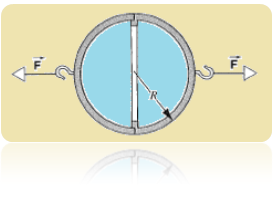Concept:-

The two brass hemispheres with an open flat can be replaced with two hemispheres with a closed flat end.

The force required to pull apart the hemispheres is equal to pressure times the surface area.

Solution:-

(a) It is given that the pressure difference between outside and inside the sphere is ?p . The radius of the circle formed by the half hemispheres which face each other is R.

Now, the surface area of the hemisphere is

A = πR2

The force required to pull the apart the brass hemisphere is

F = ?pA

= ?p (πR2) = πR2 ?p

Therefore, the force required to pull apart the two hemispheres is πR2 ?p.

(b) It is given that the inside pressure is 0.11 atm.

Thus, the pressure difference between the inside and outside of the hemispheres is

?p = 1.00 atm – 0.11 atm = 0.89 atm

Substitute 0.305 m for R and 0.89 atm for ?p in the equation F = πR2?p

F = πR2?p

= (3.14) (0.305 m)2 (0.89 atm) (1.01×105 Pa/1 atm) [(1 kg/m.s2)/1 Pa]

= (2.5997×104 kg.m/s2) [1 N/(1 kg.m/s2)] = 2.5997×104 N

Rounding off to three significant figures, the force required to pull apart the two hemispheres by the team of horses is 2.5997×104.

(c) The two teams of horses were used so as to pull apart the brass hemispheres. They could not do so as the pressure difference is too high. For such pressure difference, large amount of force is required.

_______________________________________________________________________________________________________

Problem 6:-

The below figure displays the phase diagram of carbon, showing the ranges of temperature and pressure in which carbon will crystallize either as diamond or graphite. What is the minimum depth at which diamonds can form if the local temperature is 100ºC and the subsurface rocks have density 3.1 g/cm3. Assume that, as in a fluid, the pressure is due to the weight of material lying above.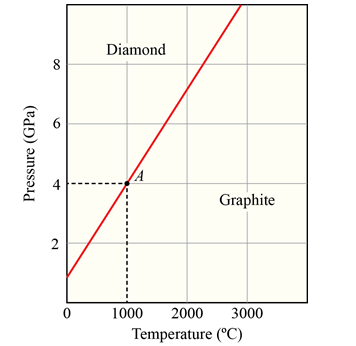Concept:-

The gauge pressure of the carbon lysing at a depth h from the surface of the Earth is

p = ρgh

Here, density of the subsurface rocks is ρ and acceleration due to gravity is g.

Solution:-

The corresponding phase diagram of carbon is represented as

From the graph, the point A gives the corresponding gauge pressure at the temperature of 1000ºC. It is found to be 4 GPa.

From the equation p = ρgh, the minimum depth at which diamonds can form at the temperature of 1000ºC is

h = p/ρg

Substitute 4 GPa for p, 3.1 g/cm3 for ρ and 9.8 m/s2 for g in the equation h = p/ρg gives

h = p/ρg

= (4 GPa) (109 Pa/1 GPa) [(1 kg/m.s2)/1 Pa] / (3.1 g/cm3) (10-3 kg/1 g) (102 cm/1 m)3 (9.8 m/s2)

= 1.3167×105 m

Rounding off to two significant figures, the minimum depth at which diamond can form from the carbon depth below the Earth is 1.3167×105 m.

___________________________________________________________________________________

Problem 7:-

In analyzing certain geological features of the Earth, it is often appropriate to assume that the pressure at some horizontal level of compensation, deep in the Earth, is the same over a large region and is equal to the exerted by the weight of the overlying material. That is, the pressure on the level of compensation is given by the hydrostatic (fluid) pressure formula. This requires, for example, that mountains have low-density roots; as shown in below figure. Consider a mountain 6.00 km high. The continental rocks have a density of 2.90 g/cm3; beneath the continent is the mantle, with a density of 3.30 g/cm3. Calculate the depth D of the root. (Hint: Set the pressure at points a and b equal; the depth y of the level of compensation will cancel out.)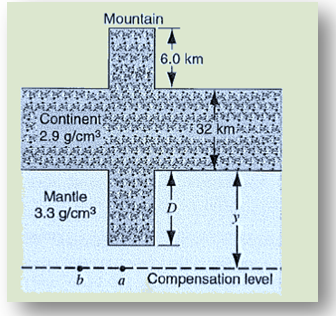Concept:-

The gauge pressure at a depth h from the surface of the Earth is

p = ρgh

Here, acceleration due to gravity is g and density of the material inside the Earth is ρ .

Solution:-

Referring the figure 15-25 given in the problem, the pressure at the point a should be considered from the top of the mountain.

So, the corresponding pressure is

pa = ρcg (6.0 km + 32 km + D) + ρMg (y – D)

Here, density of the material of the continent is ρc, density of the material of the mantle is ρM and depth of the mantle is y.

pb = ρcghc + ρMg y

Referring the figure 15-25 given in the problem, the pressure at the point b is

Here, depth of the continent is hC.

The hydrostatic pressures at the points a and b is equal.

Thus,

pa = pb

ρcghc + ρMgy = ρcg (6.0 km + 32 km + D) + ρMg (y – D)

ρchc + ρMy =  ρc (6.0 km + 32 km + D) + ρMy –ρMD

D = (ρc / ρM – ρc) [(6.0 km + 32 km) – hC]

Substitute 2.9 g/cm3 for ρc, 3.3 g/cm3 for ρM and 32 km for hC in the above equation gives

D = (ρc / ρM – ρc) [(6.0 km + 32 km) – hC]

= {[2.9 g/cm3] / [3.3 g/cm3 – 2.9 g/cm3]} [(6.0 km + 32 km) – 32 km]

= (7.25) 6.0 km) (103 m/1 km) = 43.5×103 m

Therefore, the depth D of the root is  43.5×103 m.

Related Resources:-

You might like to refer Surface Tension.

For getting an idea of the type of questions asked, refer the  Previous Year Question Papers.

```### Course Features

• 101 Video Lectures
• Revision Notes
• Previous Year Papers
• Mind Map
• Study Planner
• NCERT Solutions
• Discussion Forum
• Test paper with Video Solution